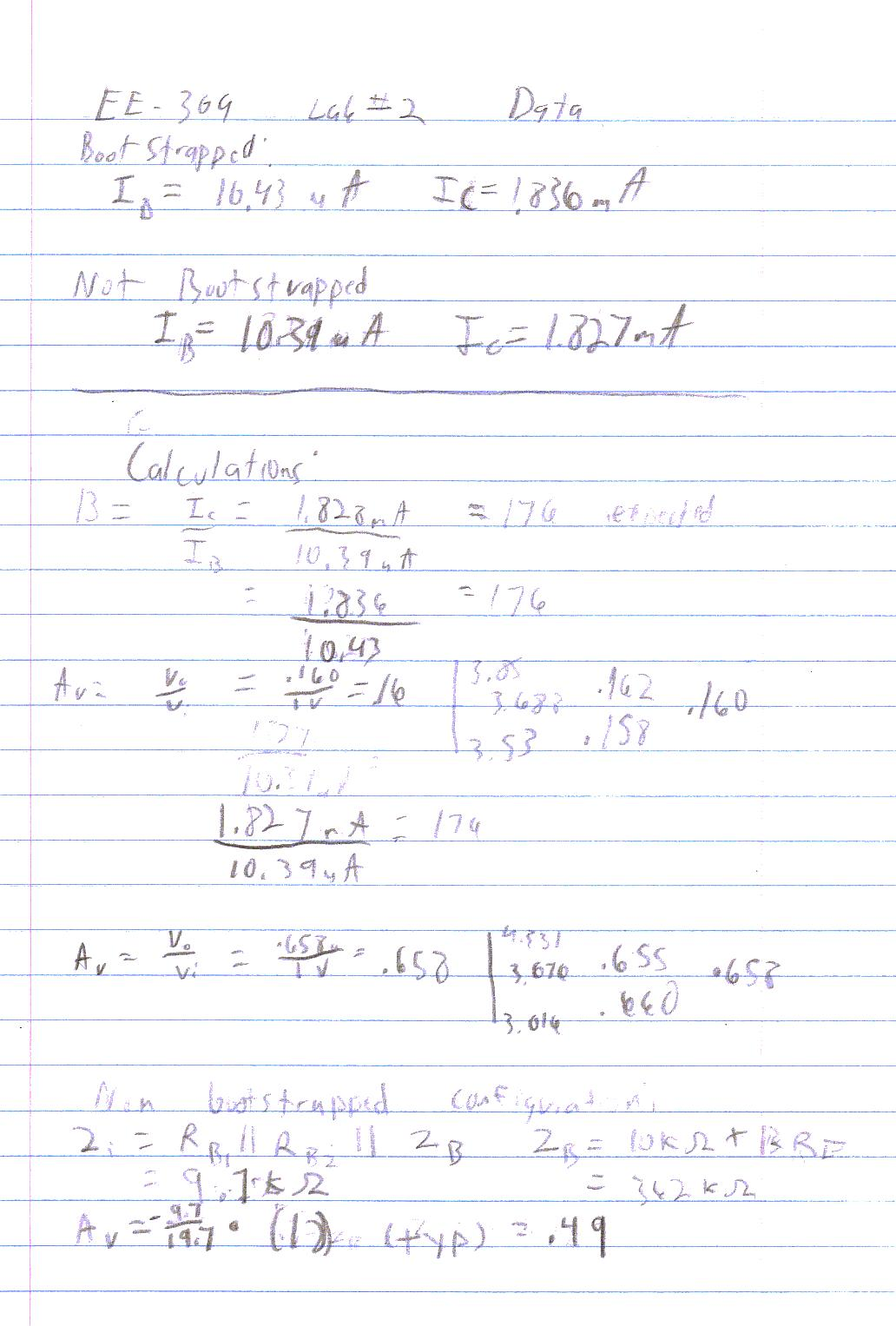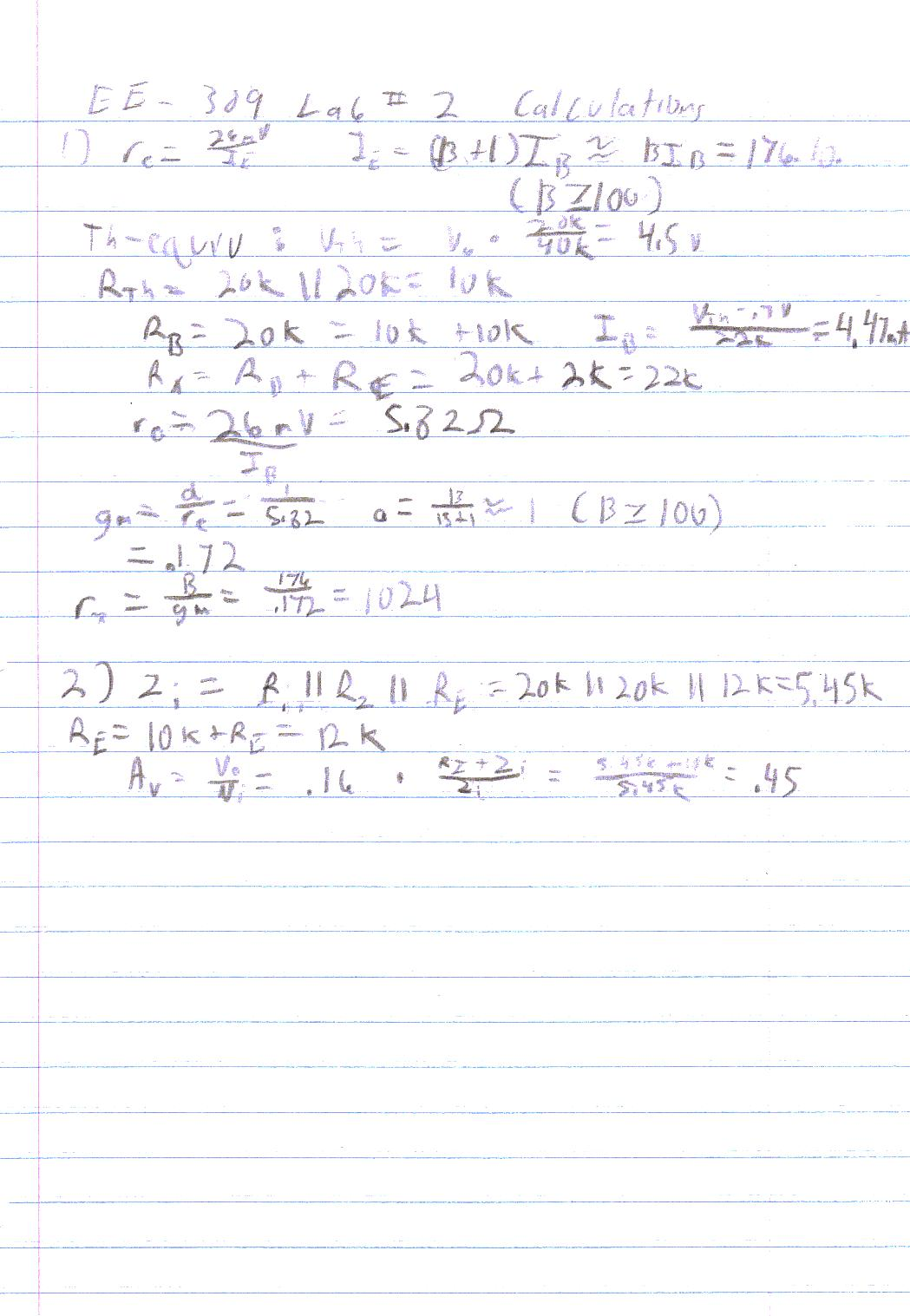EE - 309 / E01 Fall 2002

Circuit Design and Simulation

Professor: Ho

Submitted By:

Andrew Buettner

Lab #2: Bootstrapped Follower BJT Circuit

Thursday, March 06, 2003

Table Of Contents

1) Cover Page 1

2) Table of Contents 2

3) Objective 3

4) Components Used 3

5) Procedures 3

6) Lab Data / Results 3

1) Diagram 1 3

2) Diagram 2 4

3) Table 1 4

4) Diagram 3 4

5) Diagram 4 5

6) Table 2 5

7) Conclusions 5

8) Attachments 5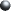Objective

The objective of this lab is to design an bootstrapped follower BJT amplifier. To make calculations on that circuit and compare them with simulated values. Then compare that circuit to a non bootstrapped version of the same circuit to find the advantages of bootstrapping.Components Used

1) PC with CircuitMaker ® installed.Procedures

1) Using CircuitMaker®, design the following circuit: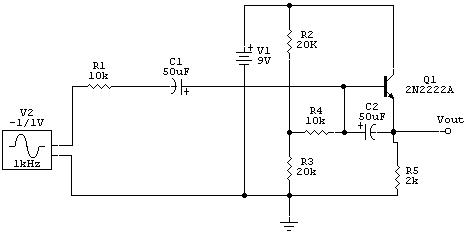2) Simulate the circuit and obtain the DC average values for base and collector current, and the voltage gain.

3) Remove C2, the bootstrapping capacitor and repeat the simulation.Lab Data / Results

1) Diagram 1: Schematic (Enlarged View)2) Diagram 2: Simulation Data from the Bootstrapped Simulation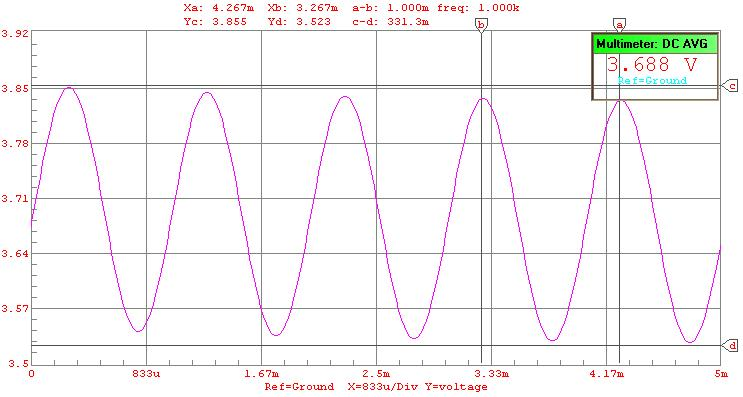3) Table 1: Analytical Data from Bootstrapped Simulation

IC

IB

Gain

b

Simulated:

1.836mA

10.43mA

0.16

176

4) Diagram 3: Non Bootstrapped Schematic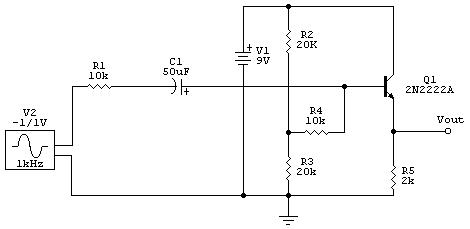5) Diagram 4: Simulation Data from the Non - Bootstrapped Mode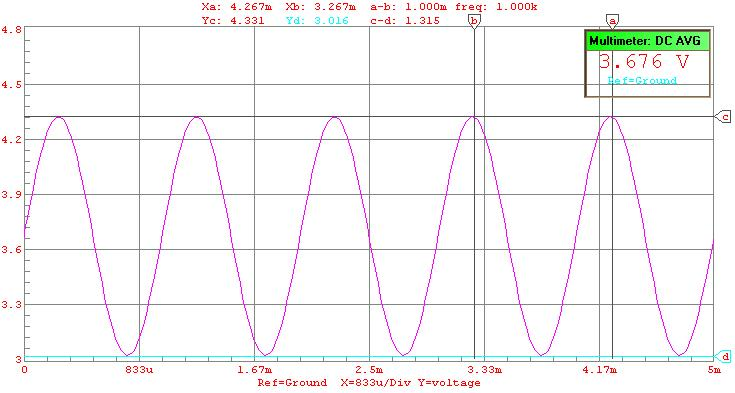6) Table 2: Analytical Data from Non - Bootstrapped Simulation

IC

IB

Gain

b

Simulated:

1.827mA

10.39mA

0.66

176Answers to Lab Questions

1) Q: What is the gm, re and rp of the transistor? Compare those to the CircuitMaker ® values.

A: gm = .172; re = 5.82W; rp = 1024. SPICE model re = 343.0mW

2) Q: Calculate the theoretical the Zi and Av of the bootstrapped circuit. Compare the values to the simulated values.

A: Zi = 5.45KW (No input resistance), 15.45KW (10KW Resistor); Av = .16

3) Q: Calculate the theoretical Zi and Av of the non bootstrapped circuit. Compare the values to the simulated values.

A: Zi = 9.7KW (No input resistance), 19.7KW (10KW Resistor); Av = 1, .49 (Input resistor). Actual Av = .66.

4) Q: Briefly describe the advantages of bootstrapping.

A: Bootstrapping increases the output impedance at the cost of voltage gain for BJT circuits. It does not, however, affect the voltage gain of MESFET based amplifiers.Conclusions

This lab has demonstrated the a bootstrapped amplifier circuit reduces the input impedance at the cost of forward gain. There were a number of values that I was unable to calculate simply because the circuit examples in the book were pertaining to FETs and not BJTs. However, I believe that the results were good, considering that I did not know what to expect. The Av of the bootstrapped circuit was based on the 10KW resistor being in series with the input. If it hadn't the gain would have been: .45. I am very uncertain of the re PSPICE model, and I do believe that to be errant, especially because of my inexperience with CircuitMaker® .Attachments

1. Original lab handout

2. Original lab data

3. Calculations